Computational & Technology Resources
an online resource for computational,
engineering & technology publications
Computational Science, Engineering & Technology Series
ISSN 1759-3158
CSETS: 5
CIVIL AND STRUCTURAL ENGINEERING COMPUTING: 2001
Edited by: B.H.V. Topping
Chapter 13

Algebraic and Combinatorial Graph Theory for Optimal Structural Analysis

A. Kaveh

Structures and Hydro-Structures Research Center, Department of Civil Engineering, Iran University of Science and Technology, Narmak, Tehran, Iran

Full Bibliographic Reference for this chapter
A. Kaveh, "Algebraic and Combinatorial Graph Theory for Optimal Structural Analysis", in B.H.V. Topping, (Editor), "Civil and Structural Engineering Computing: 2001", Saxe-Coburg Publications, Stirlingshire, UK, Chapter 13, pp 319-356, 2001. doi:10.4203/csets.5.13
Keywords: graph theory, algebraic graph theory, sparsity, well conditioned, well structured, greedy algorithm, cycle basis, ordering, Laplacian, complementary Laplacian.

Summary
For an efficient analysis of structures, the corresponding matrices should be sparse, well conditioned, and well structured. Analysis having these properties for structural matrices is called an optimal analysis, Figure 13.1. Such analysis becomes more and more important as the number of nodes and members of the structure increases.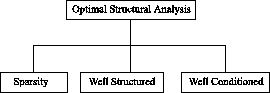Figure 13.1: Optimal structural analysis.

For optimal analysis of structures different mathematical tools are available. In this paper a brief review is provided, including the advantages and disadvantages of each tool. Best methods use powerful features of these tools in a combined form.

The engineering literature contains a number of techniques for building up large structures from smaller ones. Such techniques become important inductive tools for exploring the topological properties of structures

Historically, engineers have developed a number of rules for building up larger determinate trusses from smaller ones, and for breaking down larger trusses for analysis of their static rigidity. A critical group of methods, which involve adding and removing a single, low degree nodes are summarized by Henneberg. The idea of expansion is extended to other types of structures, and more general subgraphs are considered for addition at each step of the expansion process by Kaveh as follows: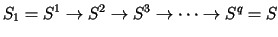(13.1)

where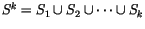and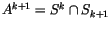.

A cycle, a cut set, a star, a planar subgraph, a repeated unit, and a subgraph with prescribed connectivity properties are examples of these employed for optimal analysis of structures. In the process of expansion restrictions can also be imposed on the intersections Ai in order to achieve additional properties required. In this article some algorithms successfully employed in optimal analysis of structures are presented. The applications are chosen from those problems which have no alternative efficient solutions.

For the formation of sparse structural matrices, graph theory can efficiently be used In here, sparsity of flexibility matrices is studied, since the sparsity of stiffness matrices can easily be achieved using the standard displacment method.

In structural engineering, one of the important sources of ill-conditioning is the use of members in a structure which have widely different stiffnesses (or flexibilities). The application of standard statical or kinematical bases (though optimal) leads to ill-conditioned structural matrices. In this paper, methods are presented for generating special cycle bases corresponding to statical bases leading to the best possible conditioning for flexibility matrices of skeletal structures.

Coarsening and uncoarsening, together with the use of the properties of the Complementary Laplacian matrices provide a powerful tool for nodal ordering of large-scale skeletal structures.

In order to transform the nodal numbering of a finite element mesh into the graph nodal ordering, ten algorithms are presented. The first six methods, order the nodes of a FEM directly, and the last four approaches use a two-step process, in which first the elements are ordered, and then ordering within elements are performed, sequentially.

For a finite element model, any of the graphs introduced in the subsequent section can be associated to transform the topological properties of the model into the connectivity properties of the selected graph. For these graphs, the complementary graphs are easily formed and the corresponding L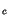matrices are constructed. Once the largest eigenvector of Lis constructed, the nodes of the graph and correspondingly the nodes of the FEM can then renumbered according to the ascending order of the entries in the eigenvector obtained.

In conclusion it should be mentioned that for optimal analysis of structures, graph theory, algebraic graph theory and matroids provide power tools for the formation of structural matrices.

purchase the full-text of this chapter (price £20)